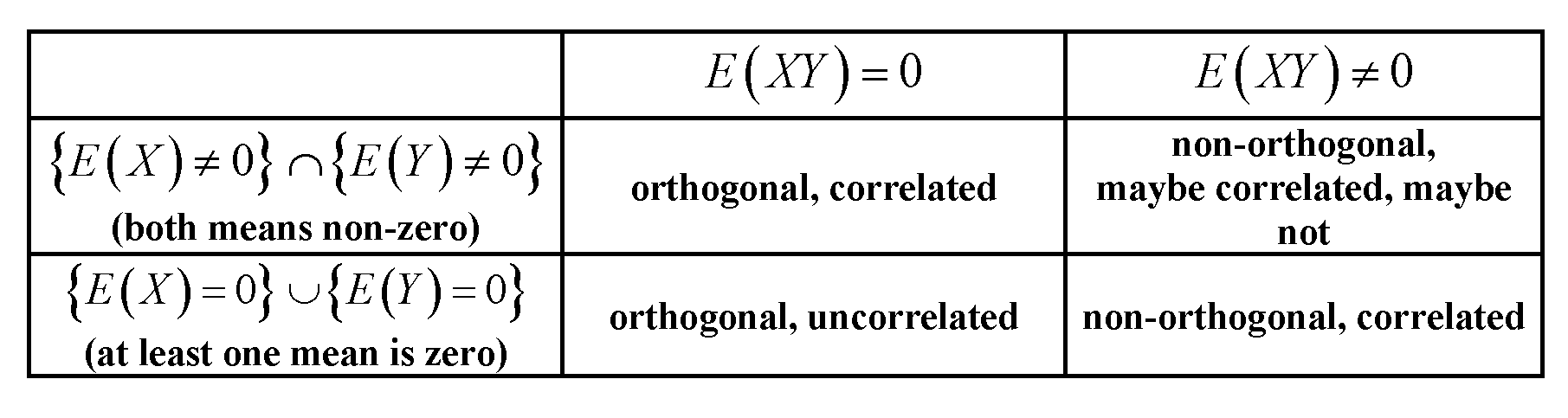### ORTHOGONALITY DOES NOT IMPLY UNCORRELATED-NESS or INDEPENDENCE:

From this post:

Orthogonality is defined as $$E[XY⋆]=0$$

So:

If $$Y=X^2$$ with symmetric pdf they they are dependent yet orthogonal.

If $$Y=X^2$$ but pdf zero for negative values, then they dependent but not orthogonal.

Therefore, orthogonality does not imply independence.

See an illustration here.

$$E[XY]$$ is the inner product of the random variables $$X$$ and $$Y$$, defined as the expectation of the product of their pdf’s: $$\langle X,Y\rangle=E[XY].$$

From this post

I realized today that orthogonality and non-correlation (in the linear sense) of two random variables $$X$$ and $$Y$$ are strongly linked in our minds – and they shouldn’t. The fact is that whether orthogonality, i.e. $$E(XY)=0$$, implies correlation, or non-correlation, depends on whether the expected values of the variables are non-zero or not. I believe in the power of tables - so here it is, and easily verifiable from the definition $$\text{Cov}(X,Y) = E(XY) – E(X)E(Y)$$:These concepts are nicely explained in this post:

Independence is a statistical concept. Two random variables $$X$$ and $$Y$$ are statistically independent if their joint distribution is the product of the marginal distributions, i.e. $f(x, y) = f(x) f(y)$ if each variable has a density $$f$$, or more generally $F(x, y) = F(x) F(y)$ where $$F$$ denotes each random variable’s cumulative distribution function.

Correlation is a weaker but related statistical concept. The (Pearson) correlation of two random variables is the expectancy of the product of the standardized variables, i.e. $\newcommand{\E}{\mathbf E} \rho = \E \left [ \frac{X - \E[X]}{\sqrt{\E[(X - \E[X])^2]}} \frac{Y - \E[Y]}{\sqrt{\E[(Y - \E[Y])^2]}} \right ].$ The variables are uncorrelated if $$\rho = 0$$. It can be shown that two random variables that are independent are necessarily uncorrelated, but not vice versa.

Orthogonality is a concept that originated in geometry, and was generalized in linear algebra and related fields of mathematics. In linear algebra, orthogonality of two vectors $$u$$ and $$v$$ is defined in inner product spaces, i.e. vector spaces with an inner product $$\langle u, v \rangle$$, as the condition that $\langle u, v \rangle = 0.$ The inner product can be defined in different ways (resulting in different inner product spaces). If the vectors are given in the form of sequences of numbers, $$u = (u_1, u_2, \ldots u_n)$$, then a typical choice is the dot product, $$\langle u, v \rangle = \sum_{i = 1}^n u_i v_i$$.

Orthogonality is therefore not a statistical concept per se, and the confusion you observe is likely due to different translations of the linear algebra concept to statistics:

1. Formally, a space of random variables can be considered as a vector space. It is then possible to define an inner product in that space, in different ways. One common choice is to define it as the covariance: $\langle X, Y \rangle = \mathrm{cov} (X, Y) = \E [ (X - \E[X]) (Y - \E[Y]) ].$ Since the correlation of two random variables is zero exactly if the covariance is zero, according to this definition uncorrelatedness is the same as orthogonality. (Another possibility is to define the inner product of random variables simply as the expectancy of the product.)

2. Not all the variables we consider in statistics are random variables. Especially in linear regression, we have independent variables which are not considered random but predefined. Independent variables are usually given as sequences of numbers, for which orthogonality is naturally defined by the dot product (see above). We can then investigate the statistical consequences of regression models where the independent variables are or are not orthogonal. In this context, orthogonality does not have a specifically statistical definition, and even more: it does not apply to random variables.

Addition responding to Silverfish’s comment: Orthogonality is not only relevant with respect to the original regressors but also with respect to contrasts, because (sets of) simple contrasts (specified by contrast vectors) can be seen as transformations of the design matrix, i.e. the set of independent variables, into a new set of independent variables. Orthogonality for contrasts is defined via the dot product. If the original regressors are mutually orthogonal and one applies orthogonal contrasts, the new regressors are mutually orthogonal, too. This ensures that the set of contrasts can be seen as describing a decomposition of variance, e.g. into main effects and interactions, the idea underlying ANOVA.

Since according to variant a), uncorrelatedness and orthogonality are just different names for the same thing, in my opinion it is best to avoid using the term in that sense. If we want to talk about uncorrelatedness of random variables, let’s just say so and not complicate matters by using another word with a different background and different implications. This also frees up the term orthogonality to be used according to variant b), which is highly useful especially in discussing multiple regression. And the other way around, we should avoid applying the term correlation to independent variables, since they are not random variables.

Rodgers et al.’s presentation is largely in line with this view, especially as they understand orthogonality to be distinct from uncorrelatedness. However, they do apply the term correlation to non-random variables (sequences of numbers). This only makes sense statistically with respect to the sample correlation coefficient $$r$$. I would still recommend to avoid this use of the term, unless the number sequence is considered as a sequence of realizations of a random variable.